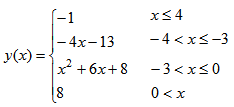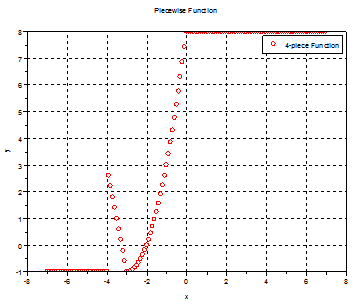# Scilab Piecewise Function

A piecewise function is a special function that has a very particular definition along the independent values. For some values of x, the function has associated a constant or a formula, but for other values of x, it has associated another formula. Each section is called a piece of the function.

In Scilab programming, there’s a number of methods to achieve this kind of functions. We could use branches (if-else code), or different cases (switch-case code), and iterations (for or while-loops) in general. In this example, we’re going to use a vectorized approach...

First, we have to understand some useful built-in functions or Scilab features for this task...

When we have a vector like this

x = -7 : 7

or

x = -7 -6 -5 -4 -3 -2 -1 0 1 2 3 4 5 6 7

we can find a range of values and a range of indices for those values.

This line

x2 = x(-4 < x & x <= -3)

is going to generate a vector of x-values that meet the condition -4 < x <= -3. Notice the particular syntax. The line uses a conditional range (extraction of values from a vector) including an ‘and’ operation (&). It gives us the x-values that meet both conditions -4 < x and x <= 3.

For the x-vector above, the result would be just one value

x2 = -3

This line

find(-4 < x & x <= -3)

is going to generate a vector of indices of the values generated before.
In this particular case, the result would be

ans = 5

because 5 is the index of the value -3 in the given vector x.
So we need to take care of both the values and the corresponding indices in a vector.

Now, let’s say that we have a 4-piece function like this one:We can design a Scilab function to perform like that described one. It’s called piecewise3.sci and accepts a vector as input:

function  y = piecewise3(x)

// first piece - a constant
y(find(x <= -4)) = -1;

// second piece - a straight line
x2 = x(-4 < x & x <= -3);
y(find(-4 < x & x <= -3)) = -4*x2 - 13;

// third piece - a parabola
x3 = x(-3 < x & x <= 0);
y(find(-3 < x & x <= 0)) = x3.^2 + 6*x3 + 8;

// fourth piece - another constant
y(find(0 < x)) = 8;

endfunction

The general idea is to find the x-values for the different pieces of the function and then apply the corresponding formula just to those values.

Finally, we have to find the correct indices in the output vector to place those values. We go one piece at a time. In the first and fourth pieces above, we don’t need to find the x-values, because the function is a constant. We just have to find the indices where the constants are going to be placed.

We can call the code from our main script or from any other sci-file, like this:

// Reset the environment
xdel(winsid()); clc; clear

// declare the function to be called
getf('piecewise3.sci');

// define your independent values in a column row
x = [-7 : .1 : 7]';

// call your previously defined function
y = piecewise3(x);

// plot
plot(x, y, 'ro')
xlabel('x'); ylabel('y');
title('Piecewise Function');
legend('4-piece Function');
xgrid

The resulting plot is this:### Another Example - Vectorization

From 'Scilab Piecewise Function' to Matlab home

From 'Scilab Piecewise Function' to Scilab Examples